# NCERT Solutions for Class 10 Science Light Reflection and Refraction Part 2## myCBSEguide App

CBSE, NCERT, JEE Main, NEET-UG, NDA, Exam Papers, Question Bank, NCERT Solutions, Exemplars, Revision Notes, Free Videos, MCQ Tests & more.

NCERT solutions for Class 10 Science Light Reflection and Refraction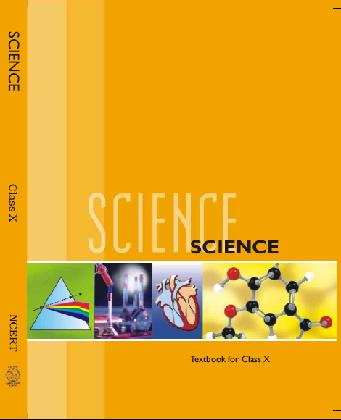NCERT Class 10 Science Chapter wise Solutions

• 1 – Chemical Reactions and Equations
• 2 – Acids, Bases and Salts
• 3 – Metals and Non-metals
• 4 – Carbon and Its Compounds
• 5 – Periodic Classification of Elements
• 6 – Life Processes
• 7 – Control and Coordination
• 8 – How do Organisms Reproduce?
• 9 – Heredity and Evolution
• 10 – Light Reflection and Refraction
• 11 – Human Eye and Colourful World
• 12 – Electricity
• 13 – Magnetic Effects of Electric Current
• 14 – Sources of Energy
• 15 – Our Environment
• 16 – Management of Natural Resources

## NCERT Solutions for Class 10 Science Light Reflection and Refraction Part 2

(a) Water

(b) Glass

(c) Plastic

(d) Clay

Ans. (d) Clay

###### 2. The image formed by a concave mirror is observed to be virtual, erect and larger than the object. Where should be the position of the object?

(a) Between the principal focus and the centre of curvature.

(b) At the centre of curvature

(c) Beyond the centre of curvature

(d) Between the pole of the mirror and its principal focus.

Ans. (d) Between the pole of the mirror and its principal focus.

###### 3. Where should an object be placed in front of convex lens to get a real image of the size of the object?

(a) At the principal focus of the lens.

(b) At twice the focal length

(c) At infinity

(d) Between the optical centre of the lens and its principal focus.

Ans. (b) At twice the focal length.

###### 4. A spherical mirror and thin spherical lens have each of focal length of -15 cm. the mirror and lens are likely to be

(a) Both concave

(b) Both convex

(c) The mirror is concave and the lens is convex

(d) The mirror is convex and lens is concave.

Ans. (a) both concave.

###### 5. No matter how far you stand from a mirror, your image appears erect. The mirror is likely to be

(a) Plane

(b) Concave

(c) Convex

(d) Either concave or convex.

Ans. (d) either plane or convex

###### 6. Which of the following lens would you prefer to use while reading small letters found in a dictionary?

(a) A convex lens of focal length 50 cm

(b) A concave lens of focal length 50 cm

(c) A convex lens of focal length 5 cm

(d) A concave lens of focal length 5 cm

Ans. (c) A convex lens of focal length 5 cm.

NCERT Solutions for Class 10 Science Light Reflection and Refraction Part 2

###### 7. We wish to obtain an erect image of an object, using a concave mirror of focal length 15 cm. What should be the range of distance of the object from mirror? What is the nature of image? Is the image larger or smaller than the object? Draw a ray diagram to show the image formation in this case.

Ans. Object must be placed in front of concave mirror between its pole and principal focus at a distance less than 15 cm.

The image formed will be virtual and erect. The size of the image is larger the object. The ray diagram is as follows: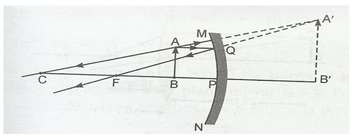NCERT Solutions for Class 10 Science Light Reflection and Refraction Part 2

###### 8. Name the type of mirror used in the following situations:

(b) Side/rear-view mirror of a vehicle.

(c) Solar furnace.

Ans. (a) Headlights of a car- concave mirror to give parallel beam of light after reflection from concave mirror.

(b) Side/rear-view mirror of vehicle- convex mirror as it forms virtual erect and diminished image to give wider view field.

(c) Solar furnace- concave mirror to concentrate sunlight to produce heat in solar furnace.

###### 9. One-half of a convex lens is covered with a black paper. Will this lens produce a complete image of the object? Verify your answers experimentally. Explain your observations.

Ans. When one-half of a convex lens is covered with a black paper, this lens produces a complete image of the object. To prove it we perform experiment: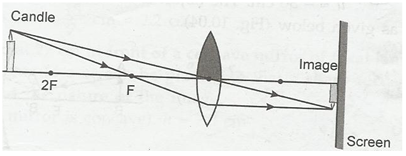Take a concave mirror and cover half part of its by using black paper. Place it vertically in a stand. On one side of it place a burning candle. On opposite side of the lens fix a white screen. Adjust the position of candle or screen till clear image of burning candle is formed on the screen. We observe that the image is complete image of the object.

From the experimental observations, we find that image formation does not depend upon the size of a lens. A similar lens can also form complete image of an object placed in front of it. However, brightness of the image decreases when some part of lens is blocked. It is because now lesser number of rays pass through the lens.

NCERT Solutions for Class 10 Science Light Reflection and Refraction Part 2

###### 10. An object 5 cm in length is held 25 cm away from a converging lens of focal length 10 cm. Draw the ray diagram and find the position, size and the nature of the image formed.

Ans. f= +10 cm, u= -25 cm and ho= 5 cm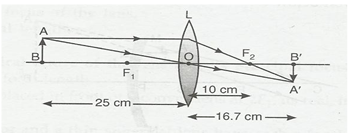1/f = 1/v – 1/u

1/v = 1/10 -1/15

1/v = 3/50

V = 50/3 cm.

The image is real and inverted at a distance of 16.7 cm from the lens on opposite side.

Magnification (m) = hi/h0= v/u

hi /5 =16.7/-25

hi = -10/3 cm. image is inverted and diminished.

NCERT Solutions for Class 10 Science Light Reflection and Refraction Part 2

###### 11. A convex lens of focal length 15 cm forms an image 10 cm from the lens. How far is the object placed from the lens? Draw the ray diagram.

Ans. f= -15 cm, v= -10 cm

1/v -1/u = 1/f

1/u = 1/15 – 1/10 = -1/30

u = -30 cm.

Ray diagram as follows: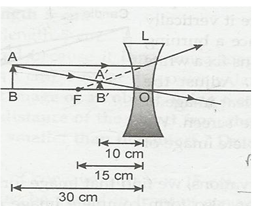NCERT Solutions for Class 10 Science Light Reflection and Refraction Part 2

###### 12. An object is placed at a distance of 10 cm from a convex mirror of focal length 15 cm. Find the position and nature of image.

Ans. f = +15 cm, u = -10 cm.

1/f = 1/v +1/u

1/v = 1/15 +1/10

1/v = 5/30

v = + 30 cm.

The image is formed 6 cm behind the mirror, it is a virtual and erect image.

NCERT Solutions for Class 10 Science Light Reflection and Refraction Part 2

###### 13. The magnification produced by a plane mirror is +1. What does this means?

Ans. m= hi/h0= v/u

Magnification produced by a plane mirror is +1 which means that size of image formed is exactly equal to size of object behind the mirror.

NCERT Solutions for Class 10 Science Light Reflection and Refraction Part 2

###### 14. An object 5.0 cm in length is placed at a distance of 20 cm in front of a convex mirror of radius of curvature 30 cm. Find the position of the image, its nature and size.

Ans. Radius of curvature (R) = 30 cm

f = R/2 = 30/2 = 15 cm

u = –20 cm, h= 5 cm.

1/v +1/u = 1/f

1/v = 1/15+ 1/20 = 7/60

v = 60/7 = 8.6 cm.

image is virtual and erect and formed behind the mirror.

hi/h0= v/u

hi/5= 8.6/20

hi = 2.2 cm.

Size of image is 2.2 cm.

NCERT Solutions for Class 10 Science Light Reflection and Refraction Part 2

###### 15. An object of size 7.0 cm is placed at 27 cm in front of a concave mirror of focal length 18 cm. At what distance from the mirror should a screen be placed, so that a sharp focused image can be obtained? Find the size and the nature of the image.

Ans. u = – 27 cm, f = – 18 cm. ho= 7.0 cm

1/v = 1/f- 1/u

1/v = -1/18 + 1/27 = -1/54

V = – 54 cm.

Screen must be placed at a distance of 54 cm from the mirror in front of it.

hi/h0= v/u

hi/h0= v/u

hi/7 = +54/-27

hi = -2 x 7 = -14 cm.

Thus, the image is of 14 cm length and is inverted image.

NCERT Solutions for Class 10 Science Light Reflection and Refraction Part 2

###### 16. Find the focal length of a lens of power -2.0 D. What type of lens is this?

Ans. Power of lens (P) = -2.0 D

P = 1/f or f = 1/m

f = 1/-2.0 = -0.5 m.

(-ve) sign of focal length means that the lens is concave lens.

NCERT Solutions for Class 10 Science Light Reflection and Refraction Part 2

###### 17. A doctor has prescribed a corrective lens of power +1.5 D. Find the focal length of the lens. Is the prescribed lens diverging or converging?

Ans. P = +1.5 D

f = 1/P = 1/+1.5 = 0.67 m.

As the power of lens is (+ve), the lens is converging lens.

## NCERT Solutions for Class 10 Science

NCERT Solutions Class 10 Science PDF (Download) Free from myCBSEguide app and myCBSEguide website. Ncert solution class 10 Science includes text book solutions from Book. NCERT Solutions for CBSE Class 10 Science have total 16 chapters. 10 Science NCERT Solutions in PDF for free Download on our website. Ncert Science class 10 solutions PDF and Science ncert class 10 PDF solutions with latest modifications and as per the latest CBSE syllabus are only available in myCBSEguide.

## CBSE app for Students

To download NCERT Solutions for class 10 Social Science, Computer Science, Home Science,Hindi ,English, Maths Science do check myCBSEguide app or website. myCBSEguide provides sample papers with solution, test papers for chapter-wise practice, NCERT solutions, NCERT Exemplar solutions, quick revision notes for ready reference, CBSE guess papers and CBSE important question papers. Sample Paper all are made available through the best app for CBSE students and myCBSEguide website.### 1 thought on “NCERT Solutions for Class 10 Science Light Reflection and Refraction Part 2”

1. in the 9th question’s answer after the diagram you have wrongly written concave instead of convex